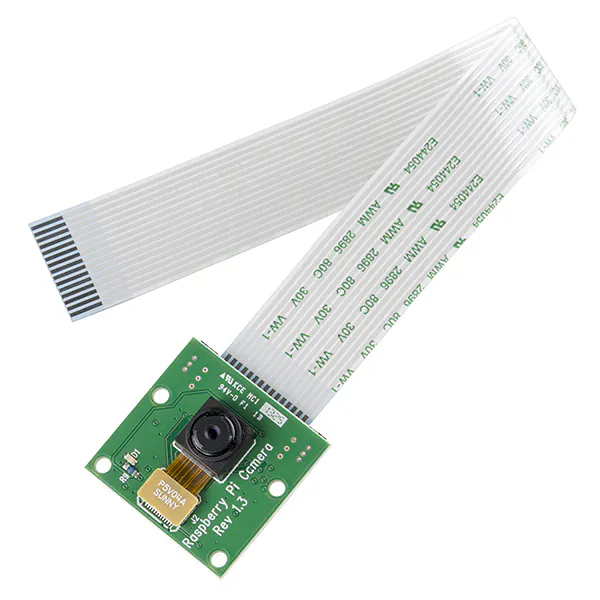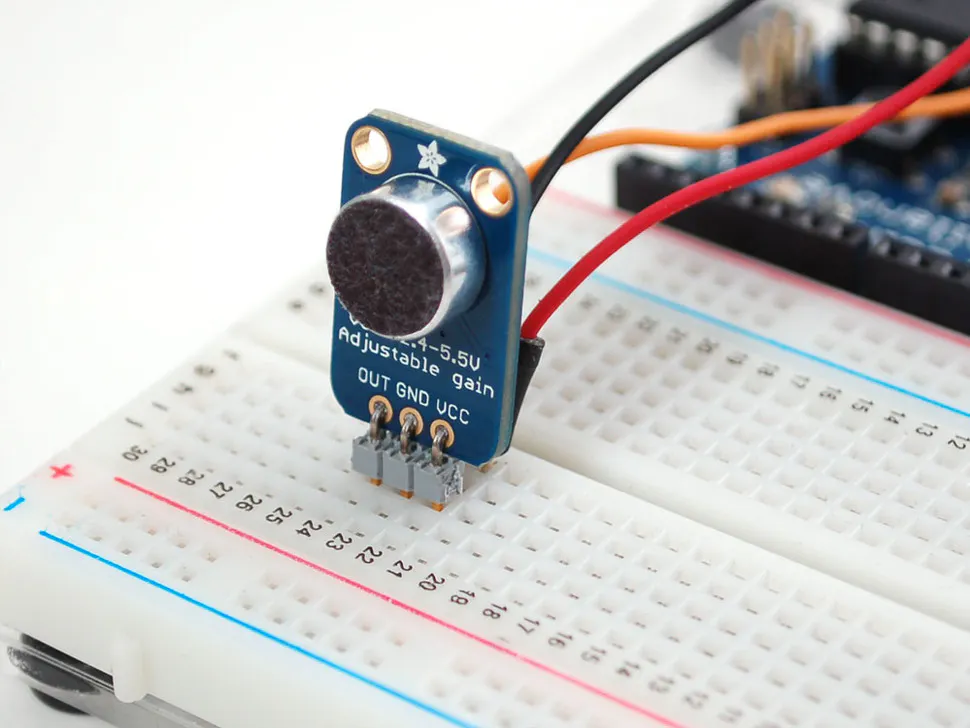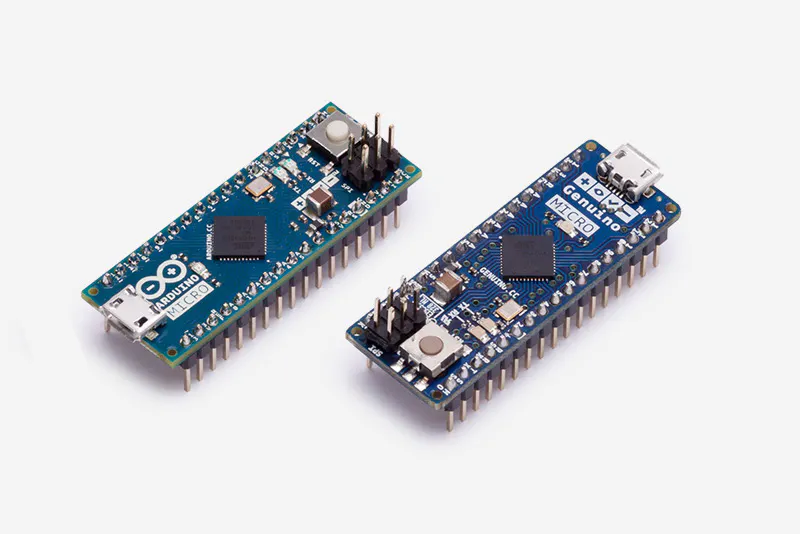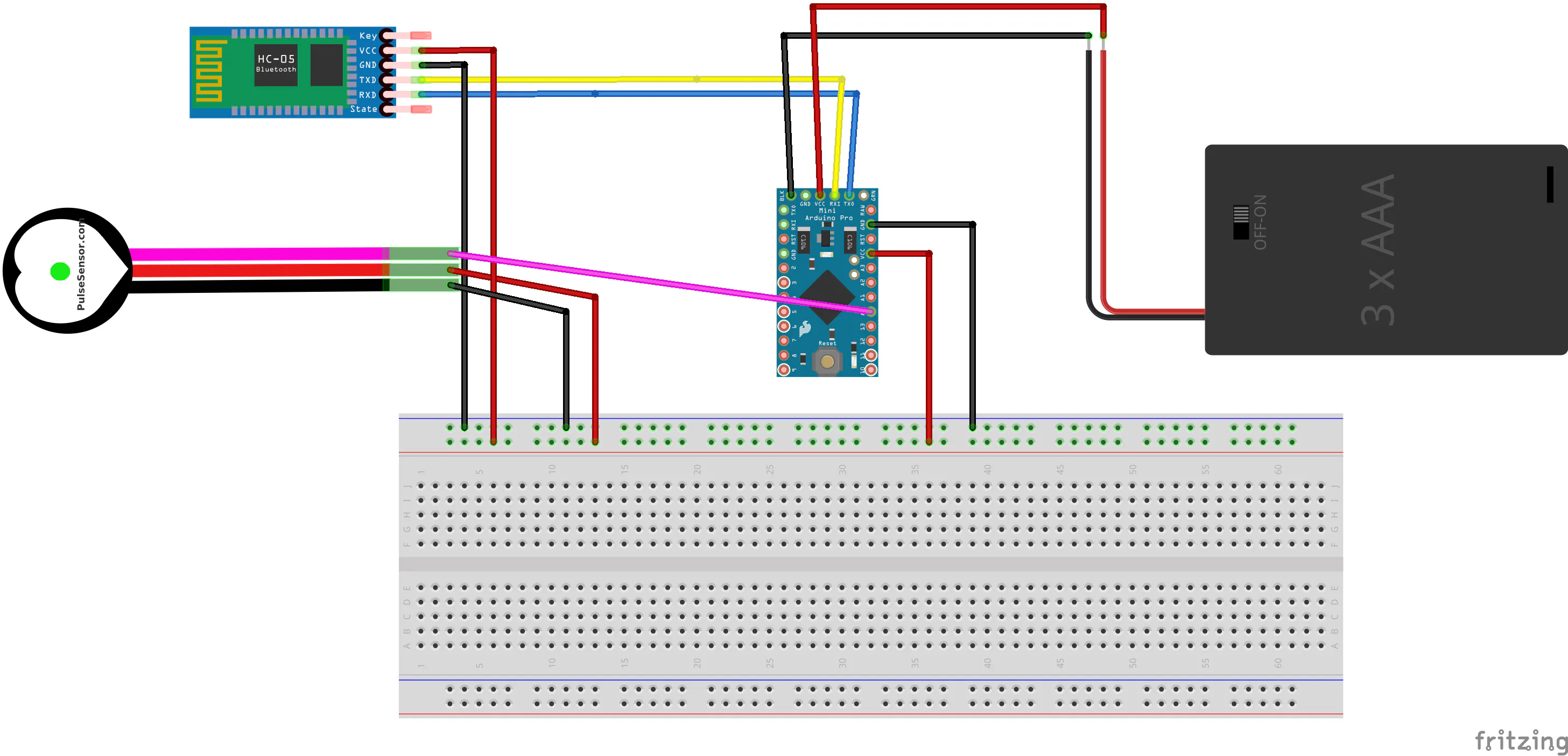# YogAI: Smart Personal Trainer

Pose estimation on a Raspberry Pi to guide and correct positions for any yogi.

## Things used in this project

### Hardware componentsRaspberry Pi 3 Model B
×1Raspberry Pi Camera Module
×1
 Monitor
×1Sonos speakers
×1
×1×1Arduino Micro & Genuino Micro
×1AA Batteries
×1HC-05 Bluetooth Module
×1

### Software apps and online servicesTensorFlowSnips AIR
 flite

### Hand tools and fabrication machines3D Printer (generic)Soldering iron (generic)

## Schematics

### heart_rate_monitor

Heart rate monitor that can pair with YogAI mirror## Code

### augment snippet

Python
example on how to augment training samples, go to https://github.com/smellslikeml/YogAI for full code
```#!/usr/bin/env python3
import pandas as pd
import numpy as np
import random
x_coords = np.array([1,0] * 14, dtype=np.int32)       # get x coordinates only
y_coords = np.flip(x_coords, axis=0)   # get y coordinates only
def rand_shift(vec, v):
return vec + v * np.random.randint(-10, 10)
def vert_swap(vec, N=96):
def col_swap(col):
return (N - col) % 96
return (vec * x_coords) + apply_vec(col_swap, (vec * y_coords))
@np.vectorize
def apply_vec(f,x):
return f(x)
out_lst = []
for row in range(df.shape):
x, y= df.iloc[row, :-1], df.iloc[row,-1]
for idx in range(5):
v = random.choice([x_coords, y_coords]) #randomly choose an index to shift
vec = rand_shift(x, v)
out_lst.append(list(vec) + [y])
#Vertical flip
if random.choice([True, False]):
v_vec = vert_swap(x)
out_lst.append(list(v_vec) + [y])
out_df = pd.DataFrame(out_lst)
out_df.to_csv('./data/yoga/augmented_poses.csv')
```

## Credits

### Salma Mayorquin

15 projects • 237 followers
Software engineer AI/ML & hardware tinkerer interested in embedded AI. Lets hack the change we want to see!

### Terry Rodriguez

15 projects • 129 followers
hack the change you want to see